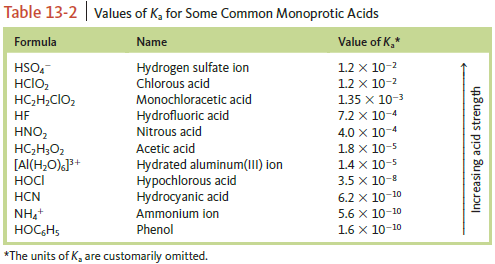Chemistry Practice Problems Ka and Kb Practice Problems Solution: Use Table 13‑2 to order the following from the str...

⚠️Our tutors found the solution shown to be helpful for the problem you're searching for. We don't have the exact solution yet.

# Solution: Use Table 13‑2 to order the following from the strongest to the weakest acid.HClO2, H2O, NH4+, HClO4

###### Problem

Use Table 13‑2 to order the following from the strongest to the weakest acid.

HClO2, H2O, NH4+, HClO4Ka and Kb

Ka and Kb

#### Q. Values of Kw as a function of temperature are as follows:a. Is the autoionization of water exothermic or endothermic?

Solved • Wed Nov 07 2018 14:50:30 GMT-0500 (EST)

Ka and Kb

#### Q. What is the ionization constant at 25 °C for the weak acid CH 3 NH3 +, the conjugate acid of the weak base CH3NH2, Kb = 4.4 × 10−4.

Solved • Mon Nov 05 2018 13:48:03 GMT-0500 (EST)

Ka and Kb

#### Q. The ionization constant for water (Kw) is 9.311 × 10−14 at 60 °C. Calculate [H3O+], [OH−], pH, and pOH for pure water at 60 °C.

Solved • Mon Nov 05 2018 12:18:03 GMT-0500 (EST)

Ka and Kb

#### Q. The ionization constant for water (Kw) is 2.9 × 10−14 at 40 °C. Calculate [H3O+], [OH−], pH, and pOH for pure water at 40 °C.

Solved • Mon Nov 05 2018 12:12:00 GMT-0500 (EST)